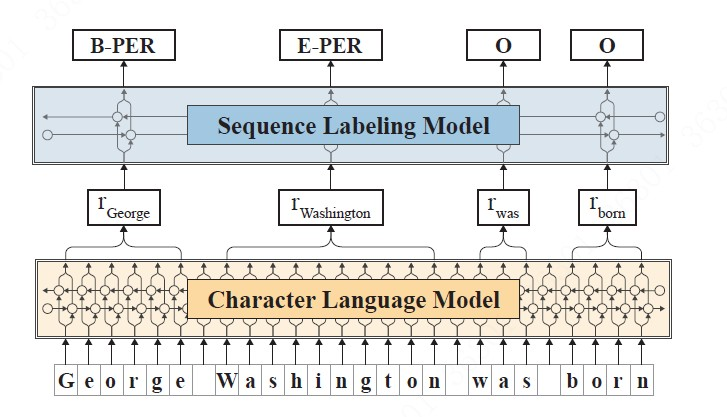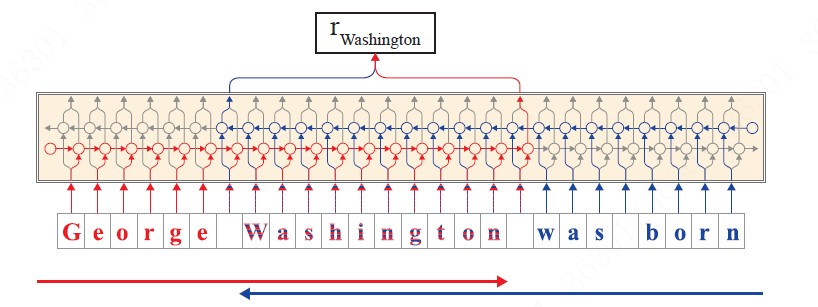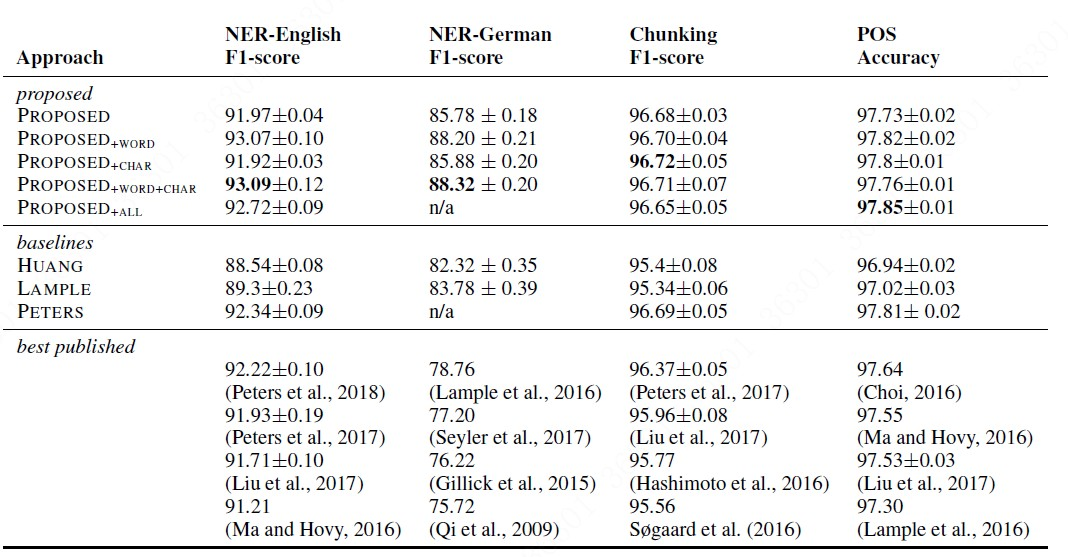• 可以在大型无标签数据集下进行训练。
• 利用上下文字符嵌入，可以更好地处理罕见和拼写错误的单词。
• 利用上下文字符嵌入，可以得到多义词的不同语境嵌入。• A. 经典embedding
• B. character-level 基于特定任务的embedding，不需要预训练，与任务的训练过程同步完成
• C. 基于上下文的，由深度LSTM各层hidden state的线性组合而成的embedding

• A. 模型以character为原子单位，在网络中，每个character都有一个对应的hidden state. – 这个特点对需要多一步分词的中文来说可能有避免分词错误导致下游function继续错误的弊端。
• B. 输出以word为单位的embedding, 这个embbeding由前向LSTM中，该词最后一个字母的hidden state 和反向LSTM中该词第一个字母的hidden state拼接组成，这样就能够兼顾上下文信息。具体说明见下图：• 在前向语言模型（红色标记），我们提取单词中最后一个字符后的输出隐藏状态作为该单词的前向表示，因为该隐藏状态包含了从句子的开头到此时传播的信息。
• 在后向语言模型（蓝色标记），我们在单词中的第一个字符之前提取输出隐藏状态作为该词的后向表示，因为，它包含了从句子末到此时传播的信息。
• 将两个隐藏状态输出拼接在一起作为该词的最终嵌入。

## 实验结果• 语料库：英文 - 10亿词语料库 德文-5亿词语料库
• 训练过程： 1个GPU跑了一周

• 10个单词左右句子产生embedding需要10ms左右，20个单词句子基本就涨到20ms，对生产环境来说勉强可以接受。

** cross entropy与 ppl的关系**

$H(u,v) = E_u[-\log v(x)] = - \sum_{x} u(x) \log v(x)$

$x$看做单词，$u(x)$为每个位置上单词的真实分布，$v(x)$为模型的预测分布$P(w_i \mid w_1, \dots ,w_{i-1})$，就可以看出 log ppl和cross entropy是等价的。唯一的区别是，由于语言模型的真实分布是未知的，因此在ppl中，真实分布用测试语料中的取样代替。即认为在给定上文$w_1,w_2,\dots ,w_{i-1}$的条件下，语料中出现单词$w_i$的概率为1，出现其他单词的概率均为0。

$u(x \mid w_1,\dots,w_{i-1}) = \begin{cases} 1, & x = w_i \\ 0, & x \neq w_i \end{cases}$

$\begin{split} H(u,v) = &-\sum_x u(x) \log v(x) \\ = & - \frac{1}{m} \sum_{i = 1}^m \lgroup \sum_{x} u(x \mid w_1,\dots, w_{i -1}) \log p(x \mid w_1, \dots,w_{i-1}) \rgroup \\ = & -\frac{1}{m} \sum_{i = 1}^m \log p(w_i \mid w_1,\dots,w_{i-1}) \\ = & \log (ppl(S)) \end{split}$